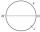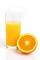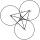V-belt

Calculate the length of the belt on pulleys with diameters of 105 mm and 393 mm at shaft distance 697 mm.

Result

l =  2.2 m

Solution:Leave us a comment of example and its solution (i.e. if it is still somewhat unclear...):Be the first to comment!To solve this example are needed these knowledge from mathematics:

Pythagorean theorem is the base for the right triangle calculator. See also our trigonometric triangle calculator.

Next similar examples:

1. CircleOn the circle k with diameter |MN| = 61 J lies point J. Line |MJ|=22. Calculate the length of a segment JN.
2. Triangle - is RT?Triangle has a circumference of 90 cm. Side b is 1 cm longer than c, side c is 31 cm longer than side a. Calculate the length of sides and determine whether triangle is a right triangle.
3. RT - inscribed circleIn a rectangular triangle has sides lengths> a = 30cm, b = 12.5cm. The right angle is at the vertex C. Calculate the radius of the inscribed circle.
4. Perimeter of triangleIn triangle ABC angle A is 60° angle B is 90° side size c is 15 cm. Calculate the triangle circumference.
5. PyramidPyramid has a base a = 5cm and height in v = 8 cm. a) calculate angle between plane ABV and base plane b) calculate angle between opposite side edges.
6. ReflectorCircular reflector throws light cone with a vertex angle 49° and is on 33 m height tower. The axis of the light beam has with the axis of the tower angle 30°. What is the maximum length of the illuminated horizontal plane?
7. KLM triangleFind the length of the sides of the triangle KLM if m = 5cm height to m = 4.5 cm and size MKL angle is 70 degrees.
8. Glass of juiceGlass of juice shaped cylinder 16 cm height and base diameter of 7 cm is filled with juice so that the level is 4 cm below the rim of the glass. Determine the maximum angle of the cup can be tilted and juice don't overflow.On a straight stretch of road is marked 12 percent drop. What angle makes the direction of the road with the horizontal plane?
10. MapleMaple peak is visible from a distance 3 m from the trunk from a height of 1.8 m at angle 62°. Determine the height of the maple.
11. Theorem proveWe want to prove the sentence: If the natural number n is divisible by six, then n is divisible by three. From what assumption we started?
12. If theIf the tangent of an angle of a right angled triangle is 0.8. Then its longest side is. .. .
13. Reference angleFind the reference angle of each angle:
14. CirclesThree circles of radius 95 cm 78 cm and 64 cm is mutually tangent. What is the perimeter of the triangle whose vertices are centers of the circles?
15. Vertices of RTShow that the points P1 (5,0), P2 (2,1) & P3 (4,7) are the vertices of a right triangle.
16. High wallI have a wall 2m high. I need a 15 degree angle (upward) to second wall 4 meters away. How high must the second wall?
17. TreeHow tall is the tree that observed in the visual angle of 52°? If I stand 5 m from the tree and eyes are two meters above the ground.